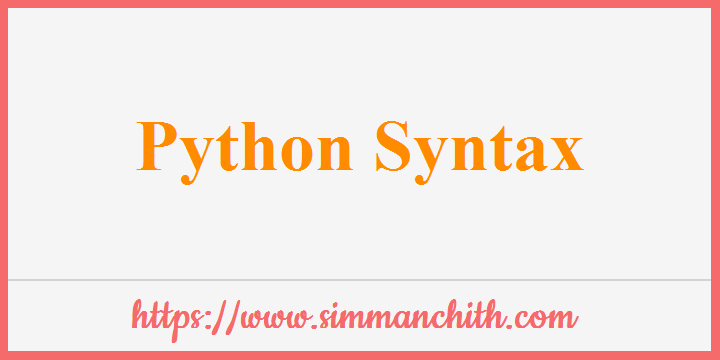# Python Syntax## Execute Python Syntax

Python syntax, as we learned on the previous page, can be carried out by typing directly in the Command Line.

``````>>> print("Hello, World!")
Hello, World!``````

Alternatively, you can create a python file on the server with the .py file extension and run it from the command line :

``C:\Users\Your Name>python myfile.py``
You can also search for these topics, Execution of Python Syntax, python syntax error invalid syntax, python execution syntax eror, what is python syntax, what is mean in python syntax, what is python syntax highlighting, what is syntax python programming, what is python syntax give examples, basic python syntax.

## Python Indentation

The spaces at the beginning of a code line are referred to as indentation.

Where as indentation in code is only for readability in other programming languages, it is critical in Python.

In order to specify a code block, Python uses the indentation.

Example 1 :- With Indentation

``````if 5 > 2:
print("Five is greater than two!")``````

Output :-

Five is greater than two!

Example 2:- Without indentation it gives syntax error :

``````if 5 > 2:
print("Five is greater than two!")``````

Output :-

File "demo_indentation_test.py", line 2
print("Five is greater than two!")
^
IndentationError: expected an indented block

Example 3 :- As a programmer, you decide number of spaces to use, but there must be at least one :

``````if 5 > 2:
print("Five is greater than two!")
if 5 > 2:
print("Five is greater than two!") ``````

Output :-

Five is greater than two!
Five is greater than two!

In the same coding block, you have to use the same number of spaces, otherwise, Python will make the mistake :

Example 4:- Syntax Error with different number of spaces in a code block :

``````if 5 > 2:
print("Five is greater than two!")
print("Five is greater than two!")``````

Output :-

File "demo_indentation2_error.py", line 3
print("Five is greater than two!")
^
IndentationError: unexpected indent

You can also search for these topics, python indentation shortcut used to error and spaces, how to check python indentation, examples, meaning, what is indentaion in python with examples, what is indentation block in python.

## Python Variables

When a value is assigned to Python, variables are created :

Example :- Variables in Python :

``````x = 5
y = "Hello, World!"
print(x)
print(y)``````

Output :-

5
Hello, World!

Python has no command for expressing a variable.

You can also search for these topics, python variables exercises,what is python variables, what is use of variable in python, what is python dummy variable, what is bad python variable name.

For the purpose of in-code documentation, Python has the capacity to comment.

Python will output the rest of the line as a comment if it begins with # :

Example :- Comments in Python :

``````#This is a comment.
print("Hello, World!")``````

Output :-

Hello, World!

You can also search for these topics, python comments symbol, syntax and block, how to write python comments, php comments starts with symbol, php commenting best practises, Example for php comments.